### Relations And Functions Class 12th Mathematics Part I CBSE Solution

##### Question 1.Let f: R → R be defined as f (x) = 10x + 7. Find the function g : R → R such that g o f = f o g = 1R.Answer:It is given that f: R → R be defined as f (x) = 10x + 7Let f(x) = f(y), where x, y ϵ R.⇒ 10x + 7 = 10y + 7⇒ x = y⇒ f is a one – one function.For y ϵ R, let y = 10x + 7.⇒ x =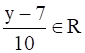Therefore, for any y ϵ R, there exists x =such that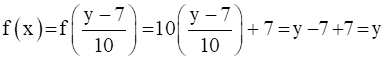⇒ f is onto.⇒ f is an invertible function.Let us define g : R → R as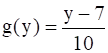Now, we get:gof(x) = g(f(x)) = g(10x + 7)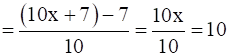And,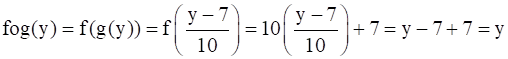⇒ gof = IR and gof = IRTherefore, the required function g : R → R is defined as.Question 2.Let f : W → W be defined as f (n) = n – 1, if n is odd and f (n) = n + 1, if n is even. Show that f is invertible. Find the inverse of f. Here, W is the set of all whole numbers.Answer:It is given that f : W → W be defined asf(n) =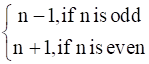Let f(n) = f(m)We can see that if n is odd and m is even, then we will have n -1 = m +1.⇒ n – m = 2⇒ this is impossibleSimilarly, the possibility of n being even and m being odd can also be ignored under a similar argument.Therefore, both n and m must be either odd or even.Now, if both n and m are odd, then we get:f(n) = f(m)⇒ n -1 = m -1⇒ n = mAgain, if both n and m are even, the we get:f(n) = f(m)⇒ n +1 = m + 1⇒ n = m⇒ f is one – one.Now, it is clear that any odd number 2r + 1 in co-domain N is the image of 2r in domain N and any even number 2r in co – domain N is the image of 2r +1 in domain N.⇒ f is onto.⇒ f is an invertible function.Now, let us define g : W → W be defined as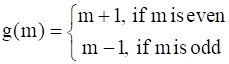Now, when n is odd:gof(n) = g(f(n)) = g (n-1) = n -1 +1 = n (when n is odd, then n-1 is even)And when n is even:gof(n) = g(f(n)) = g (n+1) = n +1 -1 = n (when n is even, then n+1 is odd)Similarly, when m is odd:fog(m) = f(g(m)) = f (m-1) = m -1 +1 = mAnd when n is even:fog(m) = f(g(m)) = f (m+1) = m +1 -1 = mTherefore, gof = IW and fog = IWTherefore, f is invertible and the inverse of f is given by f-1 = g, which is the same as f.Thus, the inverse of f is f itself.Question 3.If f : R → R is defined by f(x) = x2 – 3x + 2, find f (f (x)).Answer:It is given that f : R → R is defined by f(x) = x2 – 3x + 2.f(f(x)) = f (x2 – 3x + 2)= (x2 – 3x + 2)2 - 3(x2 – 3x + 2) + 2= x4 + 9x2 + 4 – 6x3 -12x + 4x2 – 3x2 + 9x – 6 + 2= x4 - 6x3 + 10x2 - 3xQuestion 4.Show that the function f: R → {x ∈ R : – 1 < x < 1} defined by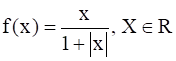is one-one and onto function.Answer:It is given that f: R → {x ∈ R : – 1 < x < 1} defined by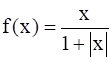, x ∈ RNow, suppose that f(x) = f(y), where x,y ϵ R.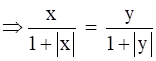We can see that if x is positive and y is negative, then we get: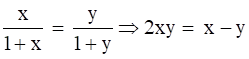Since, x is positive, and y is negative.Then, 2xy ≠ x –y.Thus, the case of x being positive and y being negative can be ruled out.Similarly, x being negative and y being positive can also be ruled out.Therefore, x and y have to be either positive or negative.When x and y are both positive, we get:f(x) = f(y)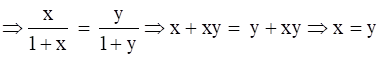And when x and y are both negative, we get:f(x) = f(y)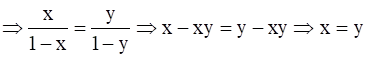⇒ f is one- one.Now, let y ϵ R such that -1 < y < 1.It y is negative, then there exists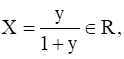such that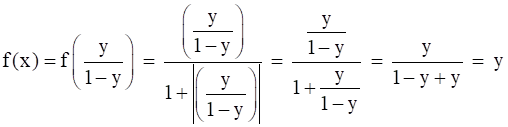⇒ f is onto.Therefore, f is one – one and onto.Question 5.Show that the function f : R → R given by f (x) = x3 is injective.Answer:Let f : R → R given by f (x) = x3.Suppose f(x) = f(y), where x, y ϵ R.⇒ x3 = y3 …(1)Now, we need to show that x = y.Suppose x ≠ y, their cubes will also not be equal.⇒ x3 ≠ y3However, this will be contraction to (1).Thus, x = yTherefore, f is injective.Question 6.Give examples of two functions f: N → Z and g: Z → Z such that g o f is injective but g is not injective.(Hint: Consider f (x) = x and g(x) = |x|).Answer:Define f: N → Z as f(x) = x and g: Z → Z as g(x) = |x|Now, we can see thatg(-1) = |-1| = 1g(1) = |1| = 1⇒ g(-1) = g(1) , but -1 ≠ 1⇒ g is not injective.Now, gof: N → Z is defined as gof(x) = g(f(x)) = g(x) = |x|Let x, y ϵ N such that gof(x) = gof(y).⇒ |x| = |y|⇒ x = yTherefore, gof is injective.Question 7.Give examples of two functions f: N → N and g : N → N such that g o f is onto but f is not onto.(Hint: Consider f (x) = x + 1 and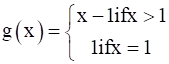Answer:It is given that f: N → N by, f(x) = x + 1And, g : N → N by,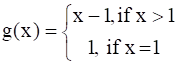Now, consider element 1 in co-domain N. So, it is clear that this element is not an image of any of the elements in domain N.⇒ f is onto.Now, gof: N → N is defined as:gof(x) = g(f(x)) = g(x+1) = (x + 1) – 1 [x ϵ N = > (x+1) > 1]Then we can see that for y ϵ N, there exists x = y ϵ N such that gof(x) = y.Therefore, gof is onto.Question 8.Given a non-empty set X, consider P(X) which is the set of all subsets of X.Define the relation R in P(X) as follows:For subsets A, B in P(X), ARB if and only if A ⊂ B. Is R an equivalence relation on P(X)? Justify your answer.Answer:We know that every set is a subset of itself, ARA for all A ϵ P(X).⇒ R is reflexive.This cannot be implied to B ⊂ A.So, if A = {1, 2} and B = {1, 2, 3}, then it cannot be implied that B is related to A.⇒ R is not symmetric.So, if ARB and BRC, then A ⊂ B and B ⊂ C.⇒ A ⊂ C⇒ R is transitive.Therefore, R is not an equivalence relation since it is not symmetric.Question 9.Given a non-empty set X, consider the binary operation ∗: P(X) × P(X) → P(X) given by A ∗ B = A ∩ B ∀ A, B in P(X), where P(X) is the power set of X. Show that X is the identity element for this operation and X is the only invertible element in P(X) with respect to the operation ∗.Answer:It is given that ∗: P(X) × P(X) → P(X) given byA ∗ B = A ∩ B ∀ A, B ϵ P(X).As we know that,⇒ A * X = A = x * A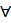A ϵ P(X).Thus, X is the identity element for the given binary operation *.Now, an element A ϵ P(X) is invertible if there exists B ϵ P(X) such thatA * B = X = B * A (As X is the identity element)A ∩ B = X = B ∩ AThis can be possible only when A = X = B.Therefore, X is the only invertible element in P(X) w.r.t. given operation *.Hence Proved.Question 10.Find the number of all onto functions from the set {1, 2, 3, ..., n} to itself.Answer:Onto function from the set {1, 2, 3, ..., n} to itself is simply a permutation on n symbols 1, 2, 3, …, n.Therefore, the total number of onto maps from {1, 2, 3, …, n} to itself is the same as the total number of permutations on n symbols 1, 2, 3, …, n, which is n!Question 11.Let S = {a, b, c} and T = {1, 2, 3}. Find F–1 of the following functions F from S to T, if it exists.F = {(a, 3), (b, 2), (c, 1)}Answer:(i) It is given that S = {a, b, c} and T = {1, 2, 3}F : S → T is defined as:F = {(a, 3), (b, 2), (c, 1)}= > F(a) = 3, F(b) = 2, F(c) = 1Therefore, F-1: T → S is given by:F-1 = {(3, a), (2, b), (1, c)}.Question 12.Let S = {a, b, c} and T = {1, 2, 3}. Find F–1 of the following functions F from S to T, if it exists.F = {(a, 2), (b, 1), (c, 1)}Answer:It is given that S = {a, b, c} and T = {1, 2, 3}F: S → T is defined as:F = {(a, 2), (b, 1), (c, 1)}= > F(b) = 1, F(c) = 1, F is not one-one.Therefore, F is not invertible⇒ F-1 does not exists.Question 13.Consider the binary operations ∗: R × R → R and o: R × R → R defined as a ∗b = |a – b| and a o b = a, ∀ a, b ∈ R. Show that ∗ is commutative but not associative, o is associative but not commutative. Further, show that ∀a, b, c ∈ R, a ∗ (b o c) = (a ∗ b) o (a ∗ c). [If it is so, we say that the operation ∗ distributes over the operation o]. Does o distribute over ∗? Justify your answer.Answer:It is given that ∗: R × R → R and o: R × R → R defined asa ∗b = |a – b| and a o b = a, ∀ a, b ∈ R.For a, b ϵ R, we get:a*b = |a-b|b*a = |b-a| = |-(a-b)| = |a-b|Therefore, a*b = b*a⇒ the operation * is commutative.We can see that(1*2)*3 = (|1-2|)*3 = |1-3| = 21*(2*3) = 1*(|2-3|) = 1*1 = 1Therefore, the operation * is not associative.Now, consider the operation o:We can observed that 1o2 = 1 and 2o1 = 2.⇒ 1o2 ≠ 2o1(where 1, 2 ϵ R)⇒ the operation o is not commutative.Let a, b, c ϵ R. Then, we get:(a o b) o c = a o c = aa o (b o c) = a o b = a⇒ (a o b) o c = a o (b o c)⇒ the operation o is associative.Now, let a, b, c ϵ R, then we have:a * (b o c) = a * b = |a –b|(a * b) o (a * c) = (|a –b|) o (|a –c| = |a –b|Thus, a * (b o c) = (a * b) o (a * c)Now,1 o (2 * 3) = 1 o (|2 –3|) = 1 o 1 = 1(1 o 2)* (1o3) = 1 * 1 = |1 –1| = 0Therefore, 1 o (2 * 3) ≠ (1 o 2)* (1o3)( where 1, 2, 3 ϵ R)Therefore, the operation o does not distribute over *.Question 14.Given a non-empty set X, let ∗: P(X) × P(X) → P(X) be defined as A * B = (A – B) ∪ (B – A), ∀A, B ∈ P(X). Show that the empty set φ is the identity for the operation ∗ and all the elements A of P(X) are invertible withA–1 = A.(Hint: (A – φ) ∪ (φ – A) = A and (A – A) ∪ (A – A) = A ∗ A = φ).Answer:It is given that ∗: P(X) × P(X) → P(X) be defined asA * B = (A – B) ∪ (B – A), A, B ∈ P(X).Now, let A ϵ P(X). Then, we get,A * ф = (A – ф) ∪ (ф – A) = A ∪ ф = Aф * A = (ф - A) ∪ (A - ф) = ф ∪ A = A⇒ A * ф = A = ф * A, A ϵ P(X)Therefore, ф is the identity element for the given operation *.Now, an element A ϵ P(X) will be invertible if there exists B ϵ P(X) such thatA * B = ф = B * A. (as ф is an identity element.)Now, we can see that A * A = (A –A) ∪ (A – A) = ф ∪ ф = фA ϵ P(X).Therefore, all the element A of P(X) are invertible with A-1 = A.Question 15.Define a binary operation ∗ on the set {0, 1, 2, 3, 4, 5} as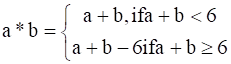Show that zero is the identity for this operation and each element a ≠ 0 of the set is invertible with 6 – a being the inverse of a.Answer:Let X = {0, 1, 2, 3, 4, 5}The operation * on X is defined as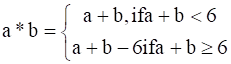An element e ϵ X is the identity element for the operation *,If a * e = a = e * aa ϵ X.For a ϵ X, we can see that:a * 0 = a + 0 = a [a ϵ X = > a + 0 < 6]0 * A = 0 + a = a [a ϵ X = > a + 0 < 6]⇒ a * 0 = a = 0 * aa ϵ X.Therefore, o is the identity element for the given operation *.An element a ϵ X is invertible if there exists b ϵ X such thata * b = 0 = b * a.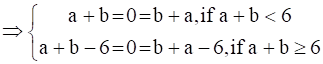a = -b or b = 6 – aBut, X = {0, 1, 2, 3, 4, 5} and a, b ϵ X. Then, a ≠ -b.Therefore, b = 6 – a is the inverse of a ϵ X.Thus, the inverse of an element a ϵ X, a ≠ 0 is 6 – a, a-1 = 6 – a.Question 16.Let A = {–1, 0, 1, 2}, B = {–4, –2, 0, 2} and f, g: A → B be functions defined by f (x) = x2 – x, x ∈ A and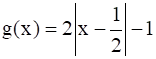, x ∈ A. Are f and g equal?Justify your answer. (Hint: One may note that two functions f: A → B and g: A → B such that f (a) = g(a) ∀ a ∈ A, are called equal functions).Answer:It is given that A = {–1, 0, 1, 2}, B = {– 4, – 2, 0, 2}And also, it is given that f, g: A → B be functions defined by f (x) = x2 – x, x ∈ A and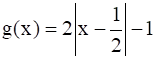, x ∈ AWe can see thatf(-1) = (-1)2 – (-1) = 1 + 1 = 2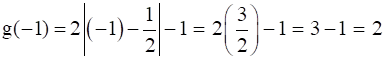⇒ f(-1) = g(-1)f(0) = (0)2 – 0 = 0 + 0 = 0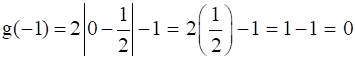⇒ f(0) = g(0)f(1) = (1)2 – 1 = 1- 1 = 0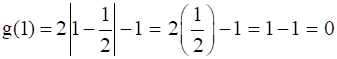⇒ f(1) = g(1)f(2) = (2)2 – 2 = 4- 2 = 2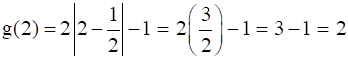f(2) = g(2)Thus, f(a) = g(a)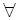. a ϵ ATherefore, the functions f and g are equal.Question 17.Let A = {1, 2, 3}. Then number of relations containing (1, 2) and (1, 3) which are reflexive and symmetric but not transitive isA. 1B. 2C. 3D. 4Answer:This is because relation R is reflexive as (1, 1), (2, 2), (3, 3) ϵ R.Relation R is symmetric as (1, 2), (2, 1) ϵ R and (1, 3), (3, 1) ϵ R.But relation R is not transitive as (3, 1), (1, 2) ϵ R but (3, 2)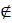R.Now, if we add any one of the two pairs (3, 2) and (2, 3) (or both) to relation R,Then, relation R will become transitive.Therefore, the total number of desired relations is one.Question 18.Let A = {1, 2, 3}. Then number of equivalence relations containing (1, 2) isA. 1B. 2C. 3D. 4Answer:It is given that A = {1, 2, 3}.An equivalence relation is reflexive, symmetric and transitive.The smallest equivalence relations containing (1, 2) is equal toR1 = {(1, 1), (2, 2), (3, 3), (1, 2), (2, 1)}Now, only four pairs are left (2, 3), (3, 2), (1, 3) and (3, 1).So, if we add one pair to R, then for symmetry we must add (3, 2).Also, for transitivity we required to add (1, 3) and (3, 1).Thus, the only equivalence relation is the universal relation.Therefore, the total number of equivalence relations containing (1, 2) is 2.Question 19.Let f: R → R be the Signum Function defined as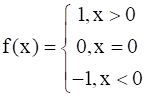and g: R → R be the Greatest Integer Function given by g(x) = [x], where [x] is greatest integer less than or equal to x. Then, does fog and gof coincide in (0, 1]?Answer:It is given thatf: R → R be the Signum Function defined as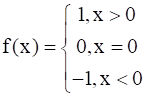Also, g: R → R is defined as g(x) = [x], where [x] is the greatest integer less than or equal to x.Now, let x ϵ (0, 1]Then, we get,[x] = 1 if x = 1 and [x] = 0 if 0 < x < 1Therefore, fog(x) = f(g(x)) = f([x]) =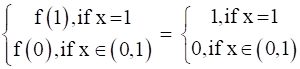gof(x) = g(f(x))= g(1) [x > 0]=  = 1Then, when x ϵ (0,1), we get fog(x) = 0 and gof(x) = 1But fog(1) ≠ gof(1)Therefore, fog and gof do not coincide in (0, 1].Question 20.Number of binary operations on the set {a, b} areA. 10B. 16C. 20D. 8Answer:A binary option * on {a, b} is a function from{a, b} × {a, b} → {a, b}i.e.yTherefore, the total number of binary operations on the set {a, b} is 24 that is 16.

PDF FILE TO YOUR EMAIL IMMEDIATELY PURCHASE NOTES & PAPER SOLUTION. @ Rs. 50/- each (GST extra)

HINDI ENTIRE PAPER SOLUTION

MARATHI PAPER SOLUTION

SSC MATHS I PAPER SOLUTION

SSC MATHS II PAPER SOLUTION

SSC SCIENCE I PAPER SOLUTION

SSC SCIENCE II PAPER SOLUTION

SSC ENGLISH PAPER SOLUTION

SSC & HSC ENGLISH WRITING SKILL

HSC ACCOUNTS NOTES

HSC OCM NOTES

HSC ECONOMICS NOTES

HSC SECRETARIAL PRACTICE NOTES

# 2019 Board Paper Solution

HSC ENGLISH SET A 2019 21st February, 2019

HSC ENGLISH SET B 2019 21st February, 2019

HSC ENGLISH SET C 2019 21st February, 2019

HSC ENGLISH SET D 2019 21st February, 2019

SECRETARIAL PRACTICE (S.P) 2019 25th February, 2019

HSC XII PHYSICS 2019 25th February, 2019

CHEMISTRY XII HSC SOLUTION 27th, February, 2019

OCM PAPER SOLUTION 2019 27th, February, 2019

HSC MATHS PAPER SOLUTION COMMERCE, 2nd March, 2019

HSC MATHS PAPER SOLUTION SCIENCE 2nd, March, 2019

SSC ENGLISH STD 10 5TH MARCH, 2019.

HSC XII ACCOUNTS 2019 6th March, 2019

HSC XII BIOLOGY 2019 6TH March, 2019

HSC XII ECONOMICS 9Th March 2019

SSC Maths I March 2019 Solution 10th Standard11th, March, 2019

SSC MATHS II MARCH 2019 SOLUTION 10TH STD.13th March, 2019

SSC SCIENCE I MARCH 2019 SOLUTION 10TH STD. 15th March, 2019.

SSC SCIENCE II MARCH 2019 SOLUTION 10TH STD. 18th March, 2019.

SSC SOCIAL SCIENCE I MARCH 2019 SOLUTION20th March, 2019

SSC SOCIAL SCIENCE II MARCH 2019 SOLUTION, 22nd March, 2019

XII CBSE - BOARD - MARCH - 2019 ENGLISH - QP + SOLUTIONS, 2nd March, 2019

# HSCMaharashtraBoardPapers2020

(Std 12th English Medium)

HSC ECONOMICS MARCH 2020

HSC OCM MARCH 2020

HSC ACCOUNTS MARCH 2020

HSC S.P. MARCH 2020

HSC ENGLISH MARCH 2020

HSC HINDI MARCH 2020

HSC MARATHI MARCH 2020

HSC MATHS MARCH 2020

# SSCMaharashtraBoardPapers2020

(Std 10th English Medium)

English MARCH 2020

HindI MARCH 2020

Hindi (Composite) MARCH 2020

Marathi MARCH 2020

Mathematics (Paper 1) MARCH 2020

Mathematics (Paper 2) MARCH 2020

Sanskrit MARCH 2020

Sanskrit (Composite) MARCH 2020

Science (Paper 1) MARCH 2020

Science (Paper 2)

Geography Model Set 1 2020-2021

MUST REMEMBER THINGS on the day of Exam

Are you prepared? for English Grammar in Board Exam.

Paper Presentation In Board Exam

How to Score Good Marks in SSC Board Exams

Tips To Score More Than 90% Marks In 12th Board Exam

How to write English exams?

How to prepare for board exam when less time is left

How to memorise what you learn for board exam

No. 1 Simple Hack, you can try out, in preparing for Board Exam

How to Study for CBSE Class 10 Board Exams Subject Wise Tips?

JEE Main 2020 Registration Process – Exam Pattern & Important Dates

NEET UG 2020 Registration Process Exam Pattern & Important Dates

How can One Prepare for two Competitive Exams at the same time?

8 Proven Tips to Handle Anxiety before Exams!